# ICSE Class 8 Maths Selina Solutions for Chapter 14 Linear Equations in One Variable

ICSE Class 8 Maths Chapter 14 Linear Equations in One Variable covers the concept of solving linear equations in one variable in contextual problems involving multiplication and division. The expressions with only one variable are called Linear Equations in one variable. Variables are the numbers mentioned in terms of alphabets. Few of the examples of linear equations in one variable are 2x = 8, 4y = 9, 3z = 7. This chapter will provide a foundation base for further complex topics related to equations.

ICSE Class 8 students should solve all the questions mentioned in the exercises to clear their doubts. By solving these exercises, students gain confidence which will help them to score good marks in their ICSE Class 8 maths examination. Students can download the ICSE Class 8 Maths Selina Solutions Chapter 14 Linear Equations in One Variable from the link provided below. The Selina Solutions are prepared by the subject experts which are explained in step by step format for easy understanding of the students.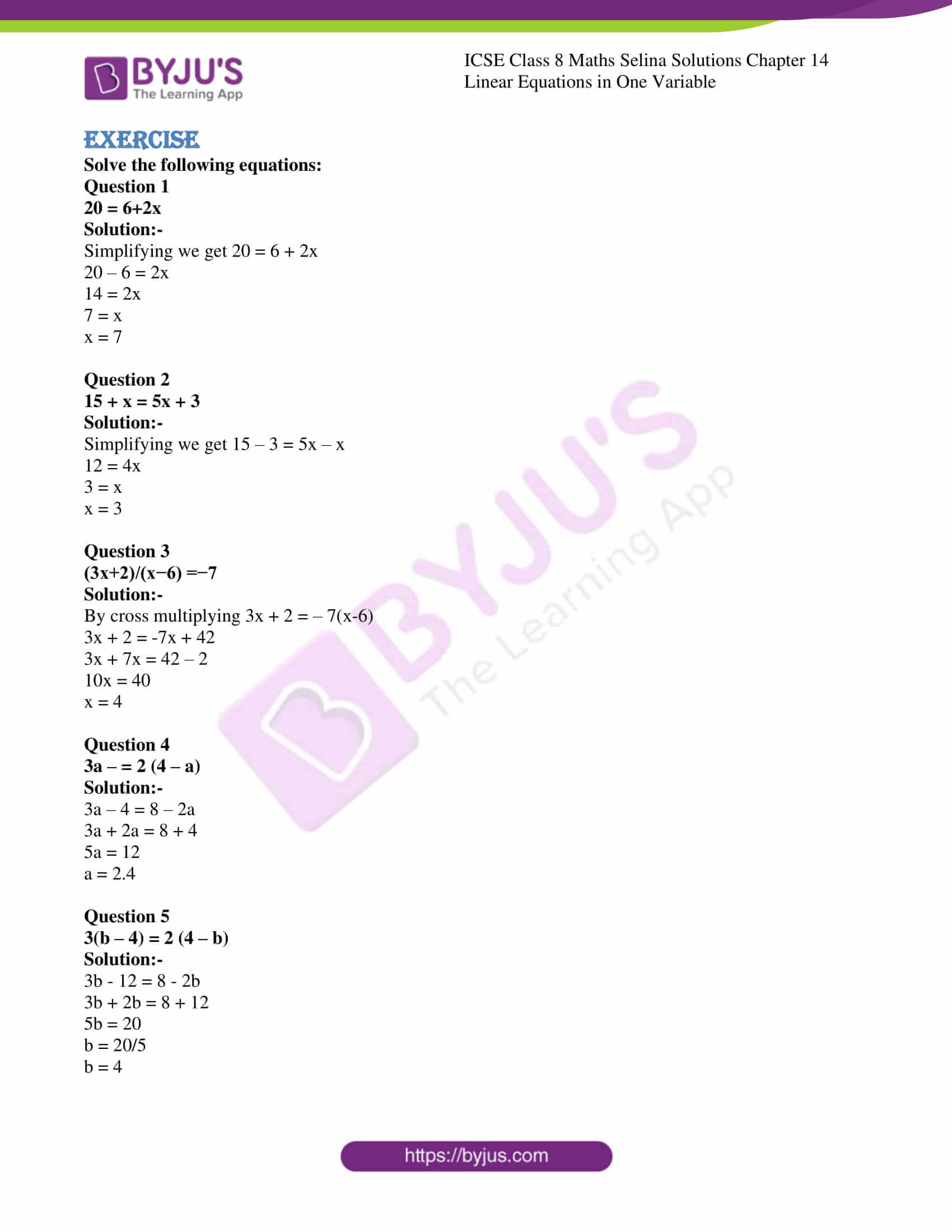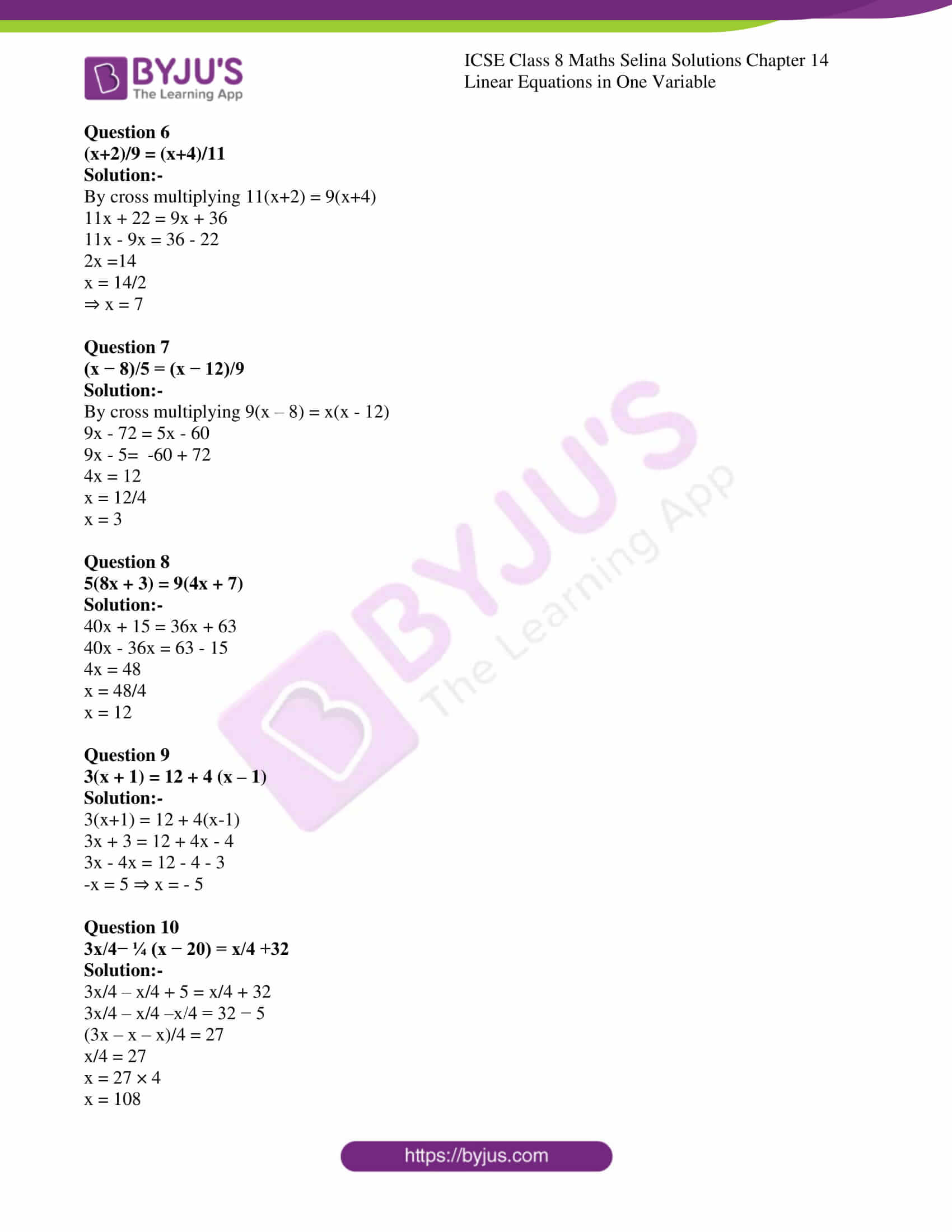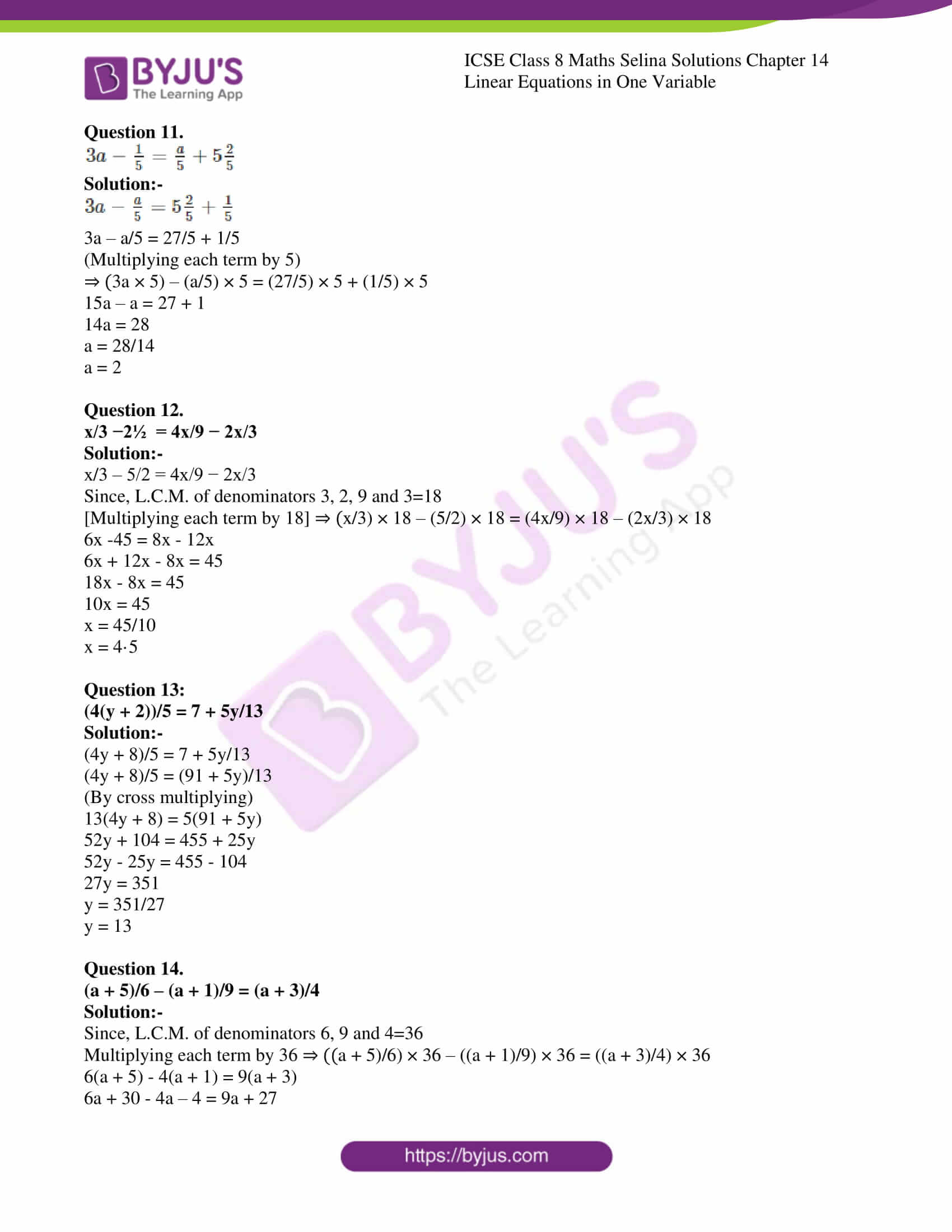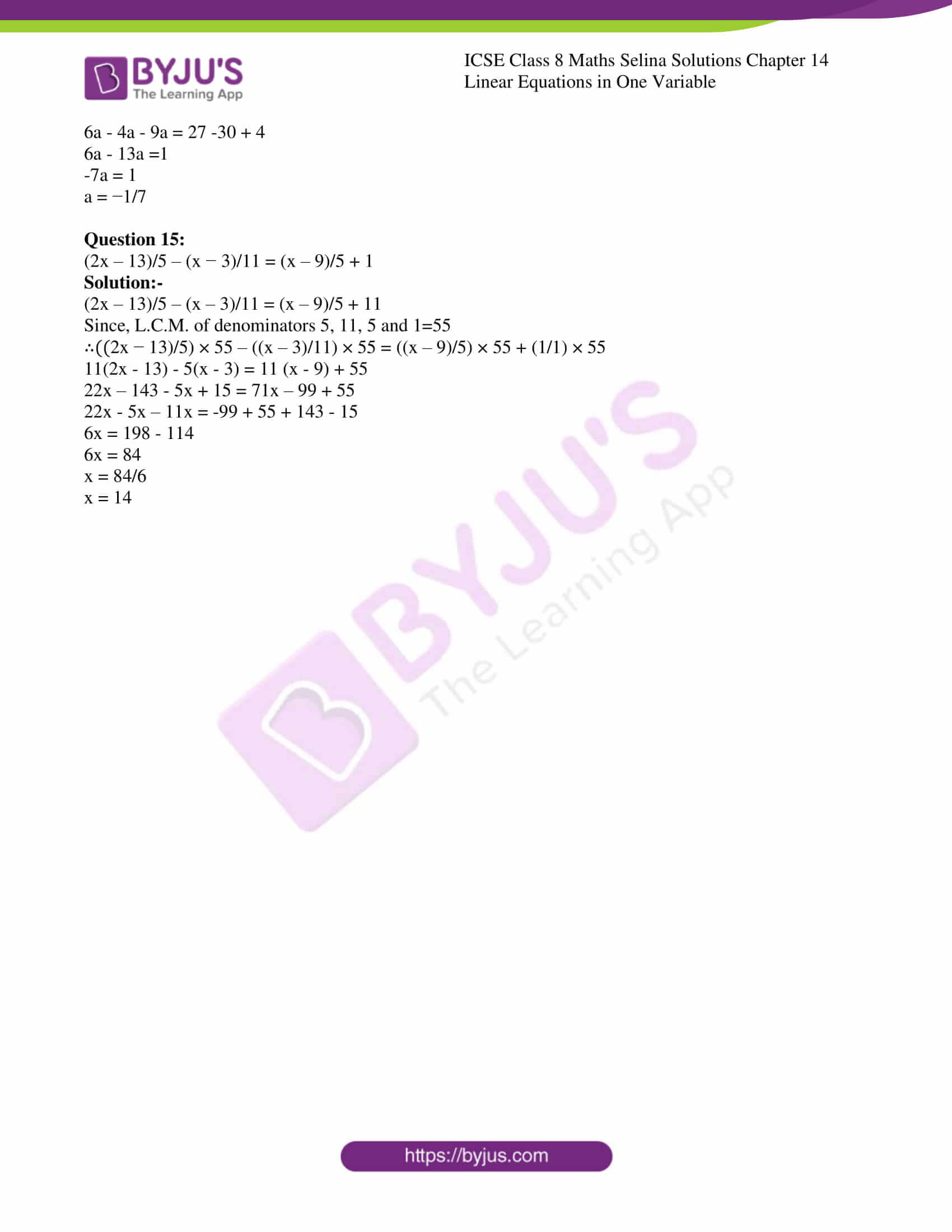ICSE Class 8 Maths Chapter 14 Linear Equations in One Variable covers a total of 15 questions and the solutions of all these questions are provided below. Students of ICSE Class 8 can also download Selina Solutions of all the other chapters for Class 8 maths subjects by clicking on ICSE Selina Class 8 Maths Solution.

### CHAPTER 14 – LINEAR EQUATIONS IN ONE VARIABLE

Exercise

Solve the following equations:

Question 1

20 = 6+2x

Solution:-

Simplifying we get 20 = 6 + 2x

20 – 6 = 2x

14 = 2x

7 = x

x = 7

Question 2

15 + x = 5x + 3

Solution:-

Simplifying we get 15 – 3 = 5x – x

12 = 4x

3 = x

x = 3

Question 3

(3x+2)/(x−6) =−7

Solution:-

By cross multiplying 3x + 2 = – 7(x-6)

3x + 2 = -7x + 42

3x + 7x = 42 – 2

10x = 40

x = 4

Question 4

3a – = 2 (4 – a)

Solution:-

3a – 4 = 8 – 2a

3a + 2a = 8 + 4

5a = 12

a = 2.4

Question 5

3(b – 4) = 2 (4 – b)

Solution:-

3b – 12 = 8 – 2b

3b + 2b = 8 + 12

5b = 20

b = 20/5

b = 4

Question 6

(x+2)/9 = (x+4)/11

Solution:-

By cross multiplying 11(x+2) = 9(x+4)

11x + 22 = 9x + 36

11x – 9x = 36 – 22

2x =14

x = 14/2

⇒ x = 7

Question 7

(x − 8)/5 = (x − 12)/9

Solution:-

By cross multiplying 9(x – 8) = x(x – 12)

9x – 72 = 5x – 60

9x – 5= -60 + 72

4x = 12

x = 12/4

x = 3

Question 8

5(8x + 3) = 9(4x + 7)

Solution:-

40x + 15 = 36x + 63

40x – 36x = 63 – 15

4x = 48

x = 48/4

x = 12

Question 9

3(x + 1) = 12 + 4 (x – 1)

Solution:-

3(x+1) = 12 + 4(x-1)

3x + 3 = 12 + 4x – 4

3x – 4x = 12 – 4 – 3

-x = 5 ⇒ x = – 5

Question 10

3x/4− ¼ (x − 20) = x/4 +32

Solution:-

3x/4 – x/4 + 5 = x/4 + 32

3x/4 – x/4 –x/4 = 32 − 5

(3x – x – x)/4 = 27

x/4 = 27

x = 27 × 4

x = 108

Question 11.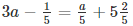Solution:-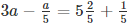3a – a/5 = 27/5 + 1/5

(Multiplying each term by 5)

⇒ (3a × 5) – (a/5) × 5 = (27/5) × 5 + (1/5) × 5

15a – a = 27 + 1

14a = 28

a = 28/14

a = 2

Question 12.

x/3 −2½ = 4x/9 − 2x/3

Solution:-

x/3 – 5/2 = 4x/9 − 2x/3

Since, L.C.M. of denominators 3, 2, 9 and 3=18

[Multiplying each term by 18] ⇒ (x/3) × 18 – (5/2) × 18 = (4x/9) × 18 – (2x/3) × 18

6x -45 = 8x – 12x

6x + 12x – 8x = 45

18x – 8x = 45

10x = 45

x = 45/10

x = 4·5

Question 13:

(4(y + 2))/5 = 7 + 5y/13

Solution:-

(4y + 8)/5 = 7 + 5y/13

(4y + 8)/5 = (91 + 5y)/13

(By cross multiplying)

13(4y + 8) = 5(91 + 5y)

52y + 104 = 455 + 25y

52y – 25y = 455 – 104

27y = 351

y = 351/27

y = 13

Question 14.

(a + 5)/6 – (a + 1)/9 = (a + 3)/4

Solution:-

Since, L.C.M. of denominators 6, 9 and 4=36

Multiplying each term by 36 ⇒ ((a + 5)/6) × 36 – ((a + 1)/9) × 36 = ((a + 3)/4) × 36

6(a + 5) – 4(a + 1) = 9(a + 3)

6a + 30 – 4a – 4 = 9a + 27

6a – 4a – 9a = 27 -30 + 4

6a – 13a =1

-7a = 1

a = −1/7

Question 15:

(2x – 13)/5 – (x − 3)/11 = (x – 9)/5 + 1

Solution:-

(2x – 13)/5 – (x – 3)/11 = (x – 9)/5 + 11

Since, L.C.M. of denominators 5, 11, 5 and 1=55

∴((2x − 13)/5) × 55 – ((x – 3)/11) × 55 = ((x – 9)/5) × 55 + (1/1) × 55

11(2x – 13) – 5(x – 3) = 11 (x – 9) + 55

22x – 143 – 5x + 15 = 71x – 99 + 55

22x – 5x – 11x = -99 + 55 + 143 – 15

6x = 198 – 114

6x = 84

x = 84/6

x = 14

### ICSE Class 8 Maths Selina Solutions Chapter 14 – Linear Equations in One Variable

Linear Equations in One Variable introduces students about the basic concepts of linear equations. It helps students to solve linear equations on one side and number on the other side. It will help the students to learn different methods to solve linear equations. The chapter also includes problems, solved and unsolved exercises which makes it more interesting and fun to learn.

Students of Class 8 ICSE can access to the solutions for Physics, Chemistry, and Biology by clicking on ICSE Class 8 Selina Solutions. Keep learning and stay tuned for further updates on ICSE and other competitive exams.